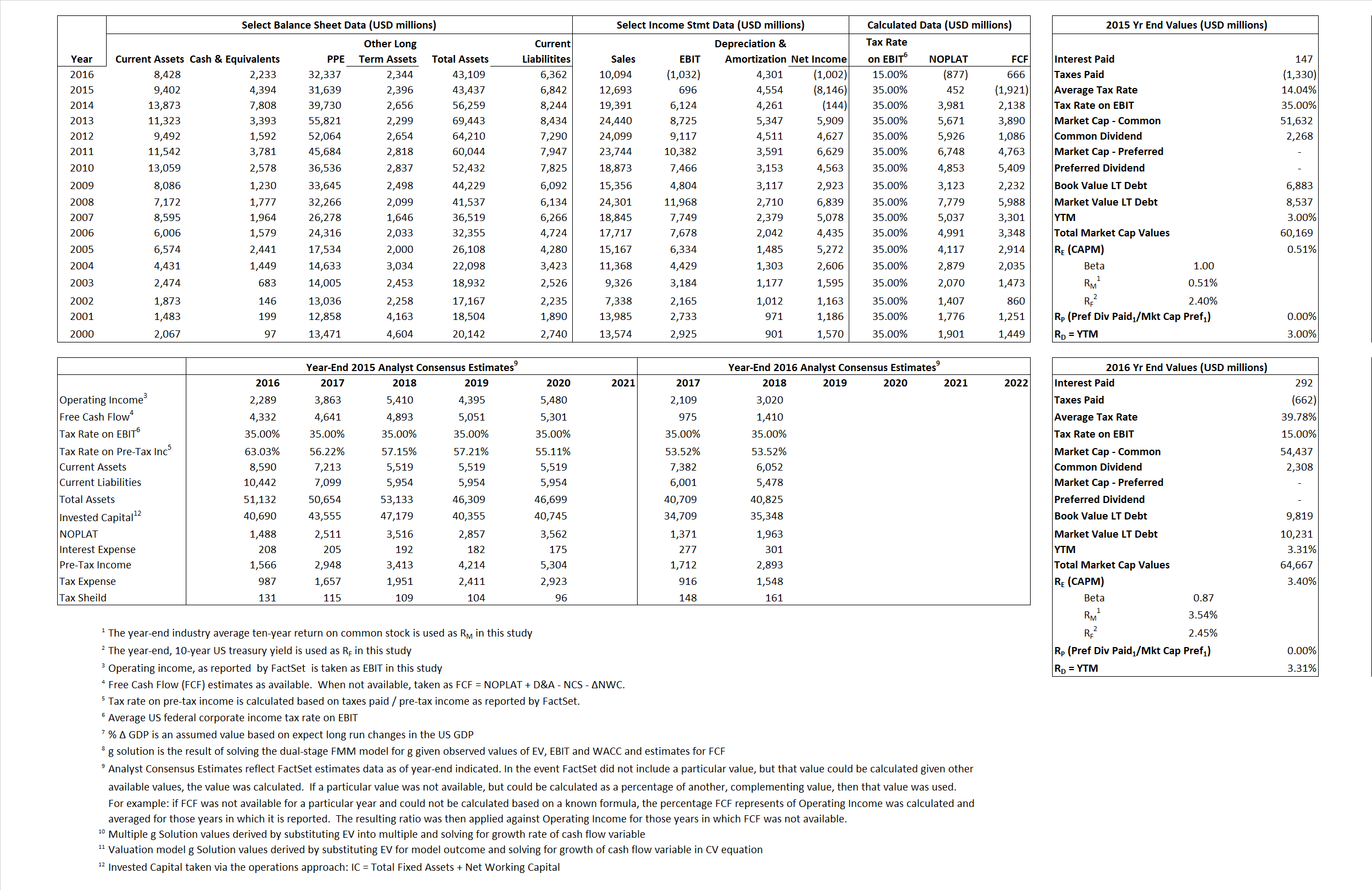# Occidental Petroleum Corp.

## Analyst Listing

The following analysts provide coverage for the subject firm as of May 2016:

 Broker Analyst Analyst Email Atlantic Equities Barry MacCarthy b.maccarthy@atlantic-equities.com Cowen & Company Charles Robertson charles.robertson@cowen.com Evercore ISI Douglas Terreson doug.terreson@evercoreisi.com Credit Suisse Edward Westlake edward.westlake@credit-suisse.com IBERIA Capital Partners Eric Fox eric.fox@iberiabank.com Piper Jaffray Guy Baber roger.read@wellsfargo.com Jefferies Jason Gammel jason.gammel@jefferies.com Tudor Pickering Holt & Co. Matthew Portillo mportillo@tphco.com Wolfe Research Paul Sankey psankey@wolferesearch.com Raymond James Pavel Molchanov pavel.molchanov@raymondjames.com Scotia Howard Weil Peter Kissel peter.kissel@scotiabank.com BMO Capital Markets Phillip Jungwirth phillip.jungwirth@bmo.com Capital One Securities Richard Tullis richard.tullis@capitalone.com GMP Securities Robert Bakanauskas bbakan@gmpsecurities.com Wells Fargo Securities Roger D. Read roger.read@wellsfargo.com Deutsche Bank Research Ryan Todd ryan.todd@db.com CRT Capital Group Tim Rezvan trezvan@sterneageecrt.com

## Primary Input Data## Derived Input Data

### Equational Form

Net Operating Profit Less Adjusted Taxes NOPLAT  452  (877)$NOPLAT\, =\, EBIT\, x\, (1 \,-\, Avg \,\,Tax\,\, Rate\,\, on\,\, EBIT)$
Free Cash Flow FCF (1,921) 666$FCF\,=NOPLAT\,+\,Non-Cash\,Expenses-\Delta NWC\,-\,NCS$
Tax Shield TS 21 116$TS\,=\,Interest\,\,Paid\,\,x\,\, Avg \,\,Tax\,\,Rate\,\, on\,\, Pre-Tax\,\, Income$
Invested Capital IC 36,595 36,747$IC\,=\,Fixed\,\,Operating\,\,Assets\,\,+\,\,Net\,\, Working\,\, Capital$
Return on Invested Capital ROIC 1.24% -2.39%$ROIC\,=\,\frac { NOPLAT }{ IC }$
Net Investment NetInv  (6,866) 4,453$NetInv\,=\,{ {IC}_{1}}-{{IC}_{0}}+Depreciation$
Investment Rate IR -1,517.68% -507.64%$IR\,=\,\frac {NetInv}{NOPLAT}$
Weighted Average Cost of Capital
WACCMarket 0.80% 3.18%$WACC\,=\,\frac { E }{ V } { R }_{ E }\,+\,\frac { P }{ V } { R }_{ P }\,+\,\frac { D }{ V } { R }_{ D }\left( 1- Avg\,\, Tax\,\,Rate\,\,on\,\,Pre-Tax\,\,Income \right)$
WACCBook     8.46%    6.57%
Enterprise value
EVMarket 55,775 62,434$EV\,=\,Market\,\,Cap\,\,Equity\,+\,\,Long\,\,Term\,\,Debt\,-\,Cash$
EVBook    56,282    62,023
Long-Run Growth
g = IR x ROIC
-18.76%   12.12% Long-run growth rates of the income variable are used in the Continuing Value portion of the valuation models.
g = %$\Delta$ GDP     2.50%    2.50%
Margin from Operations M    5.48%   -10.22%$M\,\,=\,\,\frac{EBIT}{SALES}$
Depreciation/Amortization Rate D    86.74%   131.57%$D\,\,=\,\,\frac{D+A}{EBITDA}$

## Valuation Multiple Outcomes

The outcomes presented in this study are the result of original input data, derived data, and synthesized inputs.

### model g solution

12/31/2015 12/31/2016 12/31/2015 12/31/2016 12/31/2015 12/31/2016

EV/SALES$\frac {EV}{Sales} \,= \,\frac{ROIC\, -\, g}{ROIC\,(WACC\,-\,g)}\,(1\,-\,T)\,(M)$

4.39  6.19  -.02%  11.13%  5.86%  10.06%

EV/EBITDA$\frac {EV}{EBITDA} \,= \,\frac{ROIC\, -\, g}{ROIC\,(WACC\,-\,g)}\,(1\,-\,T)\,(1\,-\,D)$

10.62 19.10 -.02% 11.13% 5.86% 10.06%

EV/NOPLAT$\frac {EV}{NOPLAT} \,= \,\frac{ROIC\, -\, g}{ROIC\,(WACC\,-\,g)}$

123.29 -71.17 -.02% 11.13% 5.86% 10.06%

EV/FCFOPS$\frac {EV}{FCF_{OPS}} \,= \,\frac{ROIC\, -\, g}{ROIC\,(WACC\,-\,g)}\,(1\,-\,T)$

-29.03 93.75 -.02% 11.13% 5.86% 10.06%

EV/EBIT$\frac {EV}{EBIT} \,= \,\frac{ROIC\, -\, g}{ROIC\,(WACC\,-\,g)}\,(1\,-\,T)$

80.14 -60.50 -.02% 11.13% 5.86% 10.06%

EV/IC$\frac {EV}{IC} \,= \,\frac{ROIC\, -\, g}{WACC\,-\,g}$

1.52 1.70 -.02% 11.13% 5.86% 10.06%